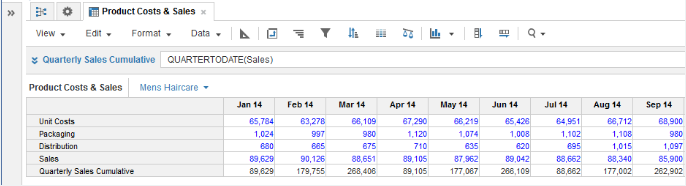1. Calculation functions
2. All Functions
3. Time and Date Functions
4. QUARTERTODATETakes a single numeric parameter and returns a cumulative sums across a quarter time span, then resets.

## Syntax

`QUARTERTODATE(x) `

where:

• x: numeric line item or a numeric value that is a function result

## Format

Input Format Output Format

x: Number

Number

## Arguments

The function uses the following arguments:

• x: Number: Numeric line item, property, or expression

## Constraints

The function has the following constraints:

• Time Scale of source must be Day, Week, or Month
• Cannot be used if model timescale is General Weeks

## Excel equivalent

• No Excel equivalent

## Example

In this example, QUARTERTODATE is used to show cumulative sales figures for each of three quarters in 2014:

`QUARTERTODATE(Sales)        `